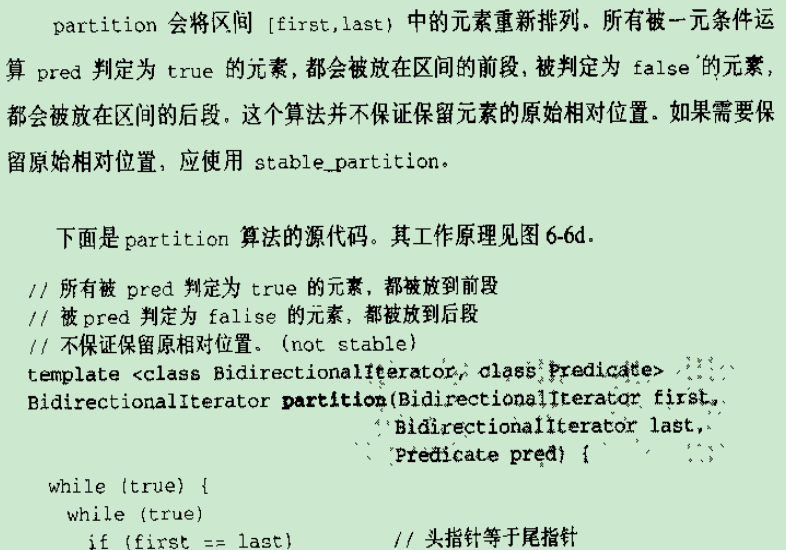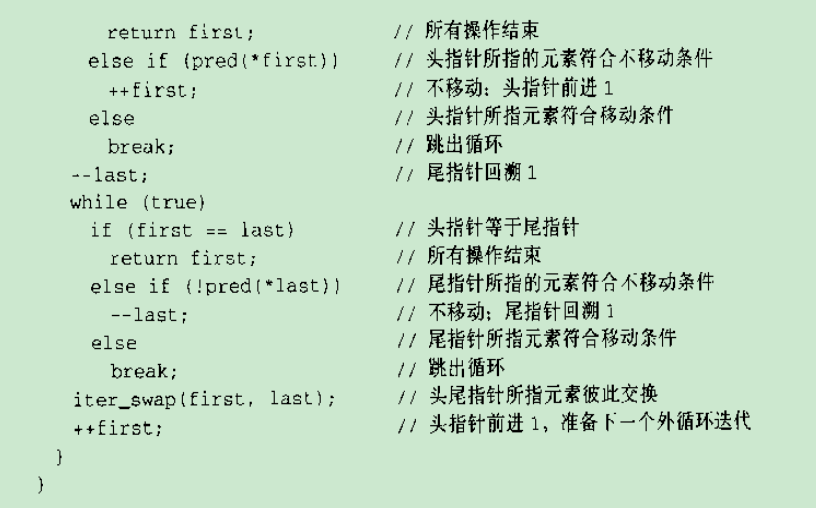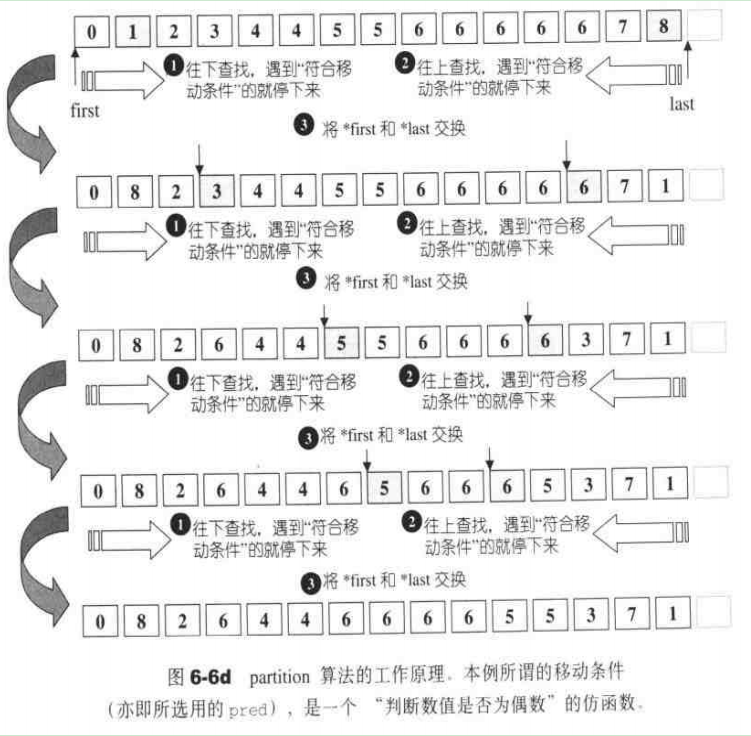• ## Partition函数

万次阅读 2017-08-17 15:06:27
快排中核心的方法应该算是Partition函数了，它的作用就是将整个数组分成小于基准值的左边，和大于基准值的右边。普通的Partition函数是这样的：public static int partition1(int[] array,int lo,int hi){ //以第一...
快排中核心的方法应该算是Partition函数了，它的作用就是将整个数组分成小于基准值的左边，和大于基准值的右边。

普通的Partition函数是这样的：

public static int partition1(int[] array,int lo,int hi){
//以第一个值为基准值，当然你也可以3取1，
int key=array[lo];
while(lo<hi){
while(array[hi]>key&&hi>lo){//从后半部分向前扫描
hi--;
}
array[lo]=array[hi];
while(array[lo]<=key&&hi>lo){//从前半部分向后扫描
lo++;
}
array[hi]=array[lo];
}
array[hi]=key;
return hi;
}

public static void main(String[] args) {
int[] a = {34,2,25,1,12,34,12,56,23,15,34,67,89,54,34};
partition2(a, 0, 14);
for(int i=0; i<a.length; i++) {
System.err.print(a[i]+" ");
}
}
打印的结果是：
34 2 25 1 12 34 12 34 23 15 **34** 67 89 54 56

看出来没，大家都是相同的基准值34是各自分开的，我们希望得结果应该是这样的：
25 1 12 12 23 15 34 34 34 34 89 54 67 56
这样的好处是相同的值，在后续的遍历过程中，不用再比较一次了。

（左程云，左神写的）
牛逼的Partition函数是这样的：

public static int[] partition1(int[] arr, int begin, int end, int pivotValue) {
int small = begin - 1;
int cur = begin;
int big = end + 1;
while (cur != big) {
if (arr[cur] < pivotValue) {
swap(arr, ++small, cur++);
} else if (arr[cur] > pivotValue) {
swap(arr, cur, --big);
} else {
cur++;
}
}
int[] range = new int;
range = small + 1;
range = big - 1;
return range;
}

public static void main(String[] args) {
int[] a = {34,2,25,1,12,34,12,56,23,15,34,67,89,54,34};
int[] b = partition1(a,0,14,34);
System.out.println(b);
System.out.println(b);
for(int i=0; i<a.length; i++) {
System.err.print(a[i]+" ");
}
}
输出的结果是：
7 //相同基准值的起始索引
10 //相同基准值的末尾索引
2 25 1 12 12 23 15 **34 34 34 34** 89 54 67 56

这样，你下一次递归直接就可以避免重复比较相同的基准值了。
展开全文快速排序
• ## partition函数

千次阅读 2015-09-08 10:14:08展开全文• Python partition函数的基本应用。partition函数主要用于拆分字符串。Python
• 比如，我们把某个集合拆分成偶数集和奇数集，partition 函数可以帮我们做到这一点： val numbers = Seq(3, 7, 2, 9, 6, 5, 1, 4, 2) //(List(2, 6, 4, 2), List(3, 7, 9, 5, 1)) numbers.partition(n => n % 2 ...
对集合进行分组
你是否尝试过将一个集合按一定的规则拆分成两个新的集合？比如，我们把某个集合拆分成偶数集和奇数集，partition 函数可以帮我们做到这一点：
val numbers = Seq(3, 7, 2, 9, 6, 5, 1, 4, 2)
//(List(2, 6, 4, 2), List(3, 7, 9, 5, 1))
numbers.partition(n => n % 2 == 0)



展开全文• 快排的递归形式 快排算法的程序结构： def quicksort(nums, l, r): if l >= r: return k = partition(nums, l, r) quicksort(nums, l, k - 1) ...其中partition函数输入参数是一个数组nums和两个位...
快排的递归形式
快排算法的程序结构：
def quicksort(nums, l, r):
if l >= r:
return
k = partition(nums, l, r)
quicksort(nums, l, k - 1)
quicksort(nums, k + 1, r)

其中partition函数输入参数是一个数组nums和两个位置索引l和r，输出为位置索引k。需要满足：

$l\le k \le r$
$nums[l..k-1]\le nums[k]$ 且$nums[k+1..r]\ge nums[k]$

而一般的partition函数的实现不仅能够满足上述两个条件，还会更严格，如把第二个条件中的其中一个等号去掉：

$nums[l..k-1] < nums[k]$ 且$nums[k+1..r]\ge nums[k]$

不但能够用来排序，还能做其它事情。
实现版本：
如下版本返回值k，均满足$nums[l..k-1] < nums[k]$ 且$nums[k+1..r]\ge nums[k]$
单向扫描版本的partition函数

for循环遍历基准元素左侧所有元素，里指针s所指元素及其左侧元素都是小于最右侧基准元素nums[r]。
出了for循环，s再前进一位，所指元素与基准元素对调，以确保最终的列表里其右侧元素均大于等于其所指元素。
for循环里以指针i为核心，其向右遍历，如果小于基准元素，则替换以大于等于基准元素。

def partition(nums, l, r):
s = l - 1
for i in range(l, r):
if nums[i] < nums[r]:
s += 1
if s != i:
nums[s], nums[i] = nums[i], nums[s]
s += 1
nums[s], nums[r] = nums[r], nums[s]
return s

双向扫描：

双重while循环出来，后有i==j且nums[l..i-1]<x，nums[i+1..r]>=x。

然后根据nums[i]与x的大小关系，分三种情况处理，并决定返回值：if nums[i] > x:
nums[i], nums[r] = x, nums[i]
elif nums[i] < x:
i += 1
if nums[i] > x:
nums[i], nums[r] = x, nums[i]


返回值i必然大于等于l，小于等于r，quicksort根据其划分的子问题规模必然小于原问题。

def partition3(nums, l, r):
x = nums[r]
i, j = l, r-1
while i < j:
while nums[i] < x and i < j: # 循环终止时i满足nums[i]>=x或者i==j
i += 1
while nums[j] >= x and i < j: # 循环终止时j满足nums[j]<x或者i==j
j -= 1
if j > i: # 说明必有nums[i]>=x且nums[j]<x，需要调换二者位置
nums[i], nums[j] = nums[j], nums[i]

if nums[i] < x:
i += 1
nums[i], nums[r] = x, nums[i]
return i

partition函数的应用
LeetCode215. 数组中的第K个最大元素

第K大，就是第L-K+1个最小元素。

class Solution(object):
def findKthLargest(self, nums, k):

if len(nums) == 0 or k <= 0 or k > len(nums):
return None
i, j = 0, len(nums)-1
k = len(nums) - k
while True:
ix = partition(nums, i, j)
if ix == k:
return nums[ix]
elif ix < k:
i = ix + 1
else:
j = ix - 1

剑指29-最小的K个数
用快排的partition方法来找到第k大的数，那么该数以及其左侧k-1个数一起，就是最小的k个数。
class Solution:
def GetLeastNumbers_Solution(self, tinput, k):
if tinput is None or len(tinput) < k or k <= 0:
return []
s, t = 0, len(tinput)-1
while True:
ix = partition(tinput, s, t)
print(s,t,ix)
if ix == k -1:
return sorted(tinput[:k])
elif ix > k -1:
t = ix -1
else:
s = ix + 1

剑指28-数组中出现次数超过一半的数字

快排的partition函数能够以某个数为基准，将数组内元素按大小分为小于该数和大于等于该数两部分。可以用来将数组分成前k个较小值和其余的值两部分，方法是迭代，将partition函数的输入区间向k和拢。
而出现次数超过一半的数，排序之后肯定会在下标len(numbers)>>1上出现，因此用partition函数找到该数，然后检查其是否满足条件即可。
每次迭代使得partition的输出更接近len(numbers)>>1这个下标，直到二者相等。如果有出现次数超过一半的数，则必然是该下标的元素。究竟是不是，也要检查一番才能确定。
注意：虽然partition的输出下标左侧的元素肯定要小于该下标所指的元素，但最终len(numbers)>>1这个下标左侧的元素却是小于等于该下标所指元素。只是由while True循环里对s和t的更新方式导致的。

class Solution:
def MoreThanHalfNum_Solution(self, numbers):

def checkMoreThanHalf(num, numbers):
half = (len(numbers)>>1)+1 # 一半加一
for n in numbers:
if n == num:
half -= 1
return half <= 0
if len(numbers) == 0:
return 0
s, t = 0, len(numbers)-1
mid = len(numbers)>>1
while True:
ix = partition(numbers, s, t)
print(s,t, ix, numbers)
if ix > mid:
t = ix - 1
elif ix < mid:
s = ix + 1
else:
break
num = numbers[mid]
return num if checkMoreThanHalf(num, numbers) else 0



展开全文• 快排扩展，Partition函数的应用问题描述问题分析python代码思维扩展   本文将介绍快排中partition函数的另一种常见的应用，如果对上篇文章感兴趣，可以点击回去看一看：快排扩展，Partition函数的应用(一)。在上一...算法 数据结构 排序算法 python
• partition函数就是快排的关键部分，作用是将数组划分成两部分，左边小于基数，右边大于基数 但实际上它也不仅仅用于快排，在求top(K)问题中也常常会用到。 下面介绍两种partition函数，他们都是双指针的方法，但具体...
• Partition函数实现的快速排序和寻找第k大数 快速排序的核心内容其实就是Partition函数，以前一直没有什么深入的理解，也只是背一下快排的代码，再后来干脆直接用sort了。但是了解Partition函数对于理解快排非常重要...快速排序
• 快排扩展，Partition函数的应用题目描述题目分析python代码扩展分析python代码牺牲空间换时间，时间O(n)，空间O(n)的算法基于排序的方法总结   快爬作为平均速度最快的一种内部排序，基础思想是基于分治的。关于...算法 数据结构 排序 快速排序 python
• partition函数为实现快速排序算法的关键函数，其作用是给定一个数组arr[]和数组中任意一个元素a，重排数组使得a左边都小于它，右边都不小于它。 public class Partition { public static int partition(int[] ...
• 使用 over 和 partition函数分组数据 除了group by， sql也可以使用 over 和partition by对结果集根据一定条件进行分组。本文解释如何结合这两个函数对数据进行分组展示。 准备数据 为了说明方便，我们定义表"...over group rank
• Partition函数有两种实现方法，单边扫描和双边扫描 Partition函数的作用就是在数组中选择一个数字，接下来把数组的数字分成两个部分，比选择的数字小的移动到数组的左边，比选择的数字大的移动到数组的右边。 单边...
• 我们现在都是被快排蒙蔽了双眼，没有意识到快最核心的划分函数Partition,当然Partition函数也就不止于快排这里，本文就从多方面来为展示Partition函数的本质和扩展作用 2.快排中的Partition及其优化： 在算法导论...
• ## python partition函数

千次阅读 2018-09-07 09:54:37
python的partition() 方法 partition() 方法用来根据指定的分隔符将字符串进行分割。 如果字符串包含指定的分隔符，则返回一个3元的元组，第一个为分隔符左边的子串，第二个为分隔符本身，第三个为分隔符右边的...
• 这个Partition函数是我在《剑指Offer》那本书上看到的，其实对应于快排是一个单项扫描。这个函数在很多算法题上都有应用，主要原理就是选定一个参考值，然后将数据分成两部分，左边都是比它小的，右边都是比它大的。...学习笔记
• 快速排序算法里的partition函数用来解决这样一个问题：给定一个数组arr[]和数组中任意一个元素a，重排数组使得a左边都小于它，右边都不小于它。 代码实现： // arr[]为数组，start、end分别为数组第一个元素和...
• 快排中partition函数非常有用, 其两种实现很难在java这种有jvm干预的情况下测出哪个更优秀, 不知道是不是测试手段比较low? 总的来说, partition2交换的次数少一下. 对算法的理解也没到化入骨髓的地步, 先备注一下. ...java
• 本文主要介绍Oracle高级查询中rank,over partition函数的用法，希望对大家有所帮助。oracle 分组 rank over
• 便准函数库定义了名为partition的算法，它接受一个谓词，对容器进行划分，使得谓词为true的值会排在容器的前半部分，而谓词为false的值会排在后半部分。算法返回一个迭代器，指向最后一个使谓词为true的元素之后的...C++
• 快速排序的精髓就在partition函数的实现。我们构建两个指针，将数组分为三部分，黑色部分全部小于pivot，中间蓝色部分都大于pivot，后面红色部分未知。i指针遍历整个数组，只要它指向的元素小于pivot就交换两个指针...
• 快速排序的核心就是partition函数，我们可以对该函数的接口作适当的改变，来运用修改过的partion函数解决一些编程算法。  这里举两个例子，这两个例子所用的partition函数如下所示： int randomRange(int start,...
• //p，r分别是这个要排序的区段的...int partition(int *arElem, int p, int r)  {  int x = arElem[r];  int i = p,j = p;  for(; i &lt; r; i++)  {  if(arElem[i] &lt; x)  {  ...
•快速排序 算法 数据结构 java

千次阅读 2014-08-08 11:34:41
add_partition，是添加磁盘分区信息的函数，负责向通用磁盘数据结构添加一个新的分区：  通用磁盘数据结构如下： struct gendisk {  int major; /* major number of driver */  ......